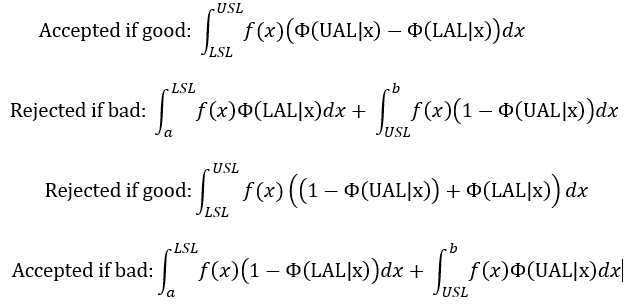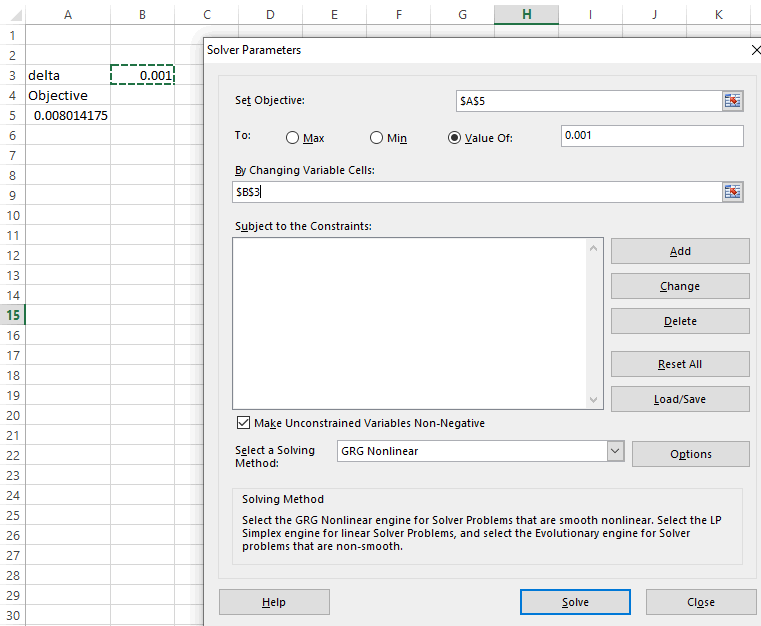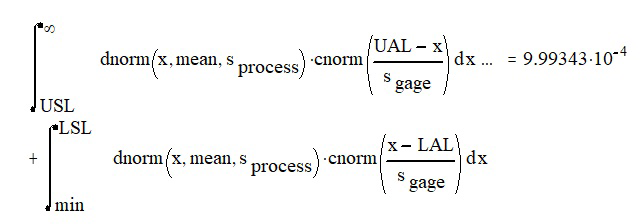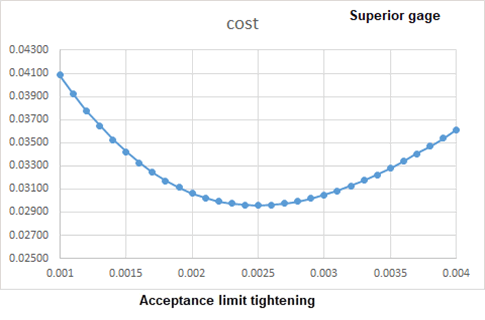#Quality Digest

Featured Product
This Week in Quality Digest Live
Metrology Features
Fred Miller
University of Arkansas Division of Agriculture leads USDA-NIFA research partnership
Daniel Croft
Noncontact scanning for safer, faster, more accurate, and cost-effective inspections
National Physical Laboratory
Using Raman spectroscopy for graphene and related 2D materials
Keith Irwin
Pros and cons of X-ray and CT techniques
Peter Büscher
Best practices for fluid sampling in cleanliness analysis
Metrology News
Allows end-users to bring 3D measurement close to the production line
Strengthens data analysis and AI capability
Makes it easy to perform all process steps, from sample observation to data analysis
General, state-specific, and courses with special requirements available
New features revolutionize metrology and inspection processes with nondimensional AI inspection
Engineering and computer science students receive new lab and learning opportunity
Supports robots from 14 leading manufacturers
Ultrasonic flaw detector now has B/C scan capability, improved connectivity, and an app to aid inspection
Tapping tooz for AR/VR competence centerBio

Metrology

## Guard Banding for Non-Capable Gages, Part 2

### Optimizing acceptance limits to minimize cost of wrong decisions

Published: Tuesday, August 24, 2021 - 12:03

All articles in this series:

Part one of this article showed that it is possible, by means of a Visual Basic for Applications program in Microsoft Excel, to calculate the fraction of in-specification product that is rejected by a non-capable gage, as well as the fraction of nonconforming product that is accepted. This calculation requires only 1) the process performance metrics, including the parameters of the distribution of the critical to quality characteristic, which need not be normal; and 2) the gage variation as assessed by measurement systems analysis (MSA).

Part 2 of the series shows how to optimize the acceptance limits to either minimize the cost of wrong decisions, or assure the customer that it will receive no more than a specified fraction of nonconforming work.

### Guard banding

We are using the attribute gage study from the AIAG reference, which involved a non-capable process with a 0.5 process performance index, i.e., a 1.5 sigma process. The specification limits are 0.45 and 0.55, respectively, which makes the process standard deviation 0.0333. I used a gage standard deviation of 0.004 to simulate the attribute gage study, and this also results in a relatively mediocre P/T ratio (24%, and the AIAG example got 24% from the signal-detection approach in its example) that will make acceptance of bad parts and rejection of good parts a problem.

Now suppose we select a lower acceptance limit (LAL) greater than the LSL, and an upper acceptance limit UAL<USL. This results in equation set 1, where ƒ(x) is the probability density function of the quality characteristic (which need not be normal), and Φ(UAL|x) is the cumulative normal probability of the measurement being less than the UAL, given actual dimension x and the gage’s standard deviation as determined from a measurement systems analysis (MSA) or gage repeatability and reproducibility study. Φ(LAL|x) is similarly the chance of the measurement being less than the LAL, given actual dimension x. Meanwhile, a and b are practical limits to stand in for negative and positive infinity, respectively, for the purposes of numerical integration. Part 1 showed how to do this with Romberg integration.

Equation set 1: Acceptance proportions for guard bandingNow suppose, for example, we move the acceptance limits 0.01 unit inside the specification limits to protect our internal or external customer from poor quality. Figure 1 shows that this will indeed reduce the fraction of bad parts that are accepted at the cost of rejecting more good ones.Figure 1: Effect of tightened acceptance limits

### Guard banding to achieve a specified nonconforming output

As we now have the means at hand to quantify the amount of good parts that are rejected and bad ones accepted, we can optimize the acceptance limits to minimize the total cost. We can alternatively select the acceptance limits to assure the customer that the total amount of nonconforming work shipped will not exceed a certain amount. The latter is the simpler scenario, so we will try this first.

We calculated previously that 0.011 (1.1%) of the process’s total output will consist of nonconforming parts that will be shipped to the internal or external customer if we rely on the actual specification limits. How much (delta) should we tighten the acceptance limits to reduce this fraction to 0.1 percent? Begin by defining an objective cell in an Excel spreadsheet as

=Gage_Integral(0.5,0.0333,0.004,0.3,0.45,0.45+delta,FALSE)+Gage_Integral(0.5,0.0333,0.004,0.55,0.7,0.55-delta,TRUE)

This is the formula for the fraction of nonconforming parts that will be accepted. The lower acceptance limit is now the LSL plus delta, and the upper acceptance limit is the USL minus delta. Figure 2 uses Excel’s Solver feature to find delta (in cell B3) to achieve the desired result. Solver determines that tightening the acceptance limits by 0.005706 will meet the customer’s requirements.Figure 2: Tighten the acceptance limits to meet customer requirement

If we add delta to the LSL and subtract it from the USL throughout, the table below confirms that 1) the conforming fractions add to the total conforming portion, and the nonconforming fractions add to the total nonconforming portion; 2) the four fractions of interest add to 1; and 3) the amount of nonconforming work shipped constitutes 0.1 percent of the total parts produced. That is, for every 1,000 parts generated, an average of one will be nonconforming and will reach the customer, and also 54 will be rejected despite being good.Result of tightened acceptance limits

MathCAD (figure 3) comes up with identical results where dnorm is the normal probability density function, and cnorm the cumulative normal probability density function. The number returned by the VBA function is, in fact, 9.99336E-04, which is almost identical to that returned by MathCAD. As a practical matter, however, the precision of the digits beyond the second or third is unlikely to be of practical significance because the values given for the process and gage standard deviation are themselves surrounded by uncertainty, as represented by their confidence intervals.Figure 3: Integration in MathCAD using Romberg integration

These results show we can live with a 1.5 sigma process; remember that 4 sigma is the generally accepted minimum for a process to be regarded as capable, if there is no alternative. We can, regardless of poor process and/or gage performance, assure our internal or external customer that it will get no more than a specified amount of nonconforming work. The price consists, of course, of the rejection of a significant quantity of good items, but we can continue to produce at this cost. The ideal is to improve the process and/or gage capability, while the ability to quantify the cost of rejection of good work and acceptance of nonconforming work enables arguments in the language of money to justify any necessary investments.

### Guard banding to minimize cost

Note that the cost of the increased protection for the customer is that 5.4 percent of the parts will be rejected even though they are good. This leads to the subject of cost minimization. Recall the assumption that the marginal cost of each part is \$1, and the cost of rework due to shipment of a nonconforming one is \$10. We can use Excel’s Solver function to optimize the acceptance limits to deliver the lowest possible cost.

Define a new objective function =D4*cost_good+D7*cost_bad, where cell D4 holds the fraction of parts that are rejected despite being good, and D7 the fraction of the parts that are accepted despite being bad. Figure 4 uses Excel’s Solver function to minimize the total cost, with delta = 0.00466. (The figure 0.005706 from the previous example was used as the initial guess.)Figure 4: Minimization of guard banding cost

Figure 5 shows graphically that 0.00466 does minimize the total cost to about 0.0623 per part.Figure 5: Cost of guard banding, dollars per part manufactured

### We’re going to need a better gage

The ability to quantify the cost of an inadequate P/T ratio can also be used to make a case, in the language of money, for better metrology equipment and/or improvement of the process capability. The original problem statement used a gage standard deviation of 0.004. Suppose a more expensive gage is available that has a standard deviation of only 0.002 units. If we replace 0.004 with 0.002 in the function’s arguments, e.g., =Gage_Integral(0.5,0.0333,0.002,0.45,0.5,0.45+delta,FALSE), the new solution is delta = 0.00250 rather than the previous 0.00466. That is, the acceptance limits need to be only 0.00250 rather than 0.00466 inside the specification limits to minimize the cost. Figure 6 shows that the total cost of lack of gage precision is now slightly less than 0.03 per part manufactured, rather than 0.0623.Figure 6: Benefits of a superior gage

We know, of course, that less equipment variation is better, but now we can make the argument in the language of money. The cost of equipment variation is 0.032 less per part with the superior gage. If we are making only 100 parts, then the better gage will probably cost a lot more than the \$3.20 we can save by using it. If the customer needs 100,000 parts, then a potential saving of \$3,200 is a good incentive to spend, for example, \$100 on a better gage. The need for a better gage becomes much greater as the marginal cost of the parts and/or the cost of external failure increase.

### Summary

This article has shown that it is possible to calculate for a given set of tightened acceptance limits the proportion of good parts that a gage will reject, and bad parts that the gage will accept, given the statistical distribution of the underlying process and the gage’s own equipment variation. This offers remedies for situations in which the gage capability (i.e., precision/tolerance ratio) is not adequate, and also enables economic decisions as to whether a superior gage should be purchased if available.

### References

1. Automotive Industry Action Group. Measurement Systems Analysis, 4th Edition, Section C, 2010.

### Discuss